### Showing videos fromEddie Woo with a total of 3,931 videos

06:32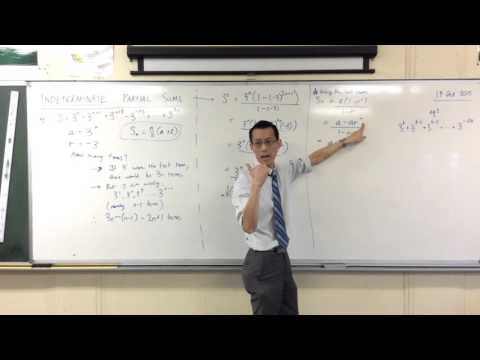Indeterminate Partial Sums - Alternative Methods (1 of 2: Using the last term)
3y4m ago
06:46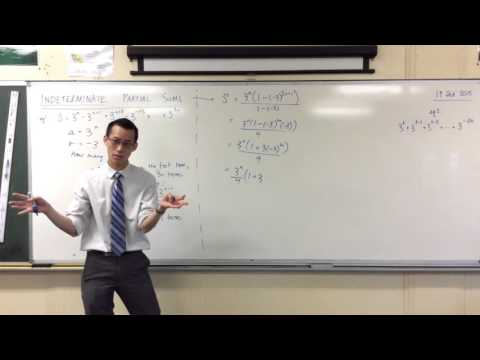Indeterminate Partial Sums (2 of 2: Using the sum formula)
3y4m ago
12:05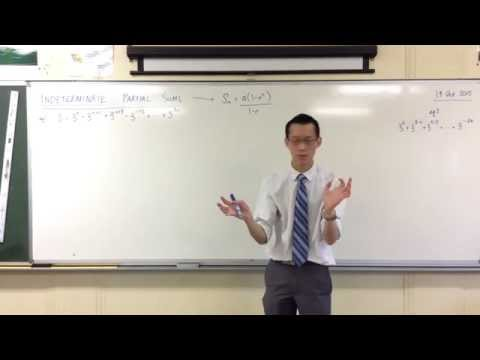Indeterminate Partial Sums (1 of 2: Finding the length of the series)
3y4m ago
06:38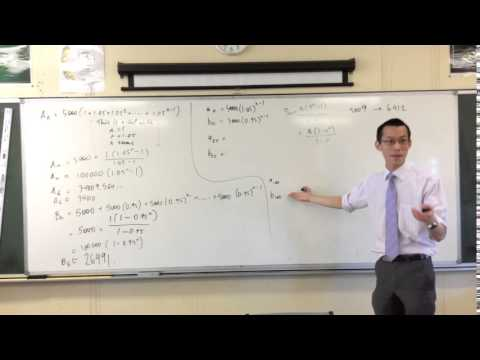Application of Series Example Question (3 of 3: Interpreting/Solving)
3y4m ago
11:25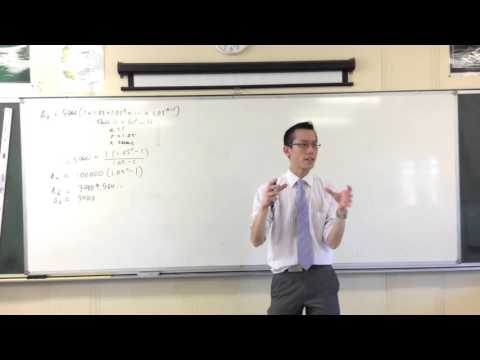Application of Series Example Question (2 of 3: Generalising the Series)
3y4m ago
13:43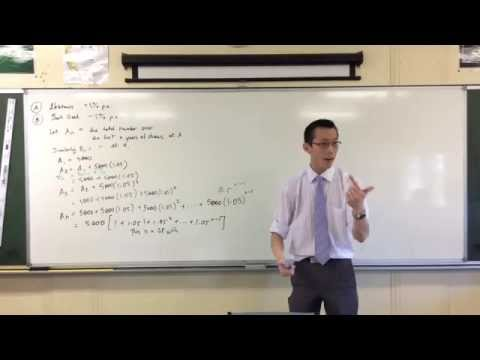Application of Series Example Question (1 of 3: Setting up the equations)
3y4m ago
07:17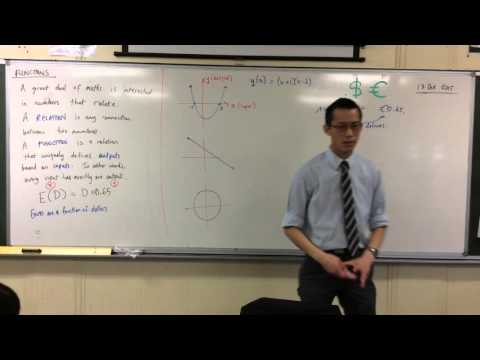Introduction to Functions (2 of 2: Examples & Counter-Examples)
3y4m ago
07:08Introduction to Functions (1 of 2: Basic Idea & Formal Definition)
3y4m ago
07:27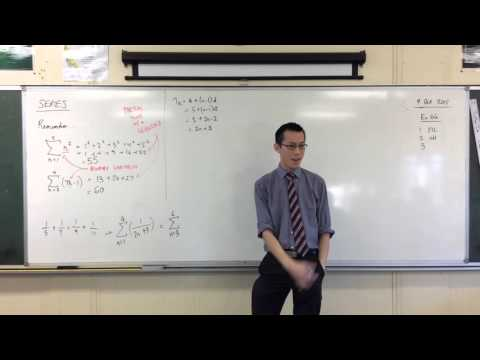Introduction to Series (2 of 2: Constructing Sigma Notation)
3y5m ago
06:03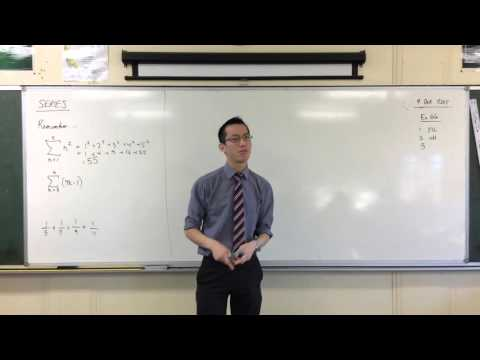Introduction to Series (1 of 2: Interpreting Sigma Notation)
3y5m ago
03:32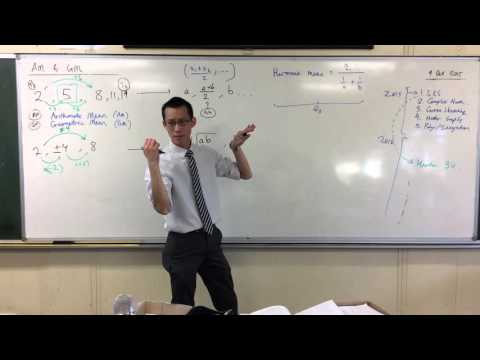Arithmetic & Geometric Mean (2 of 2: Inserting Multiple Means)
3y5m ago
09:26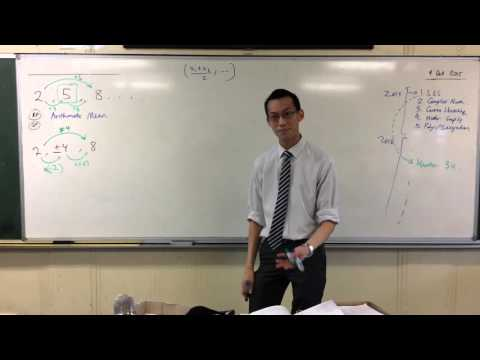Arithmetic & Geometric Mean (1 of 2: Introductory Example)
3y5m ago
08:24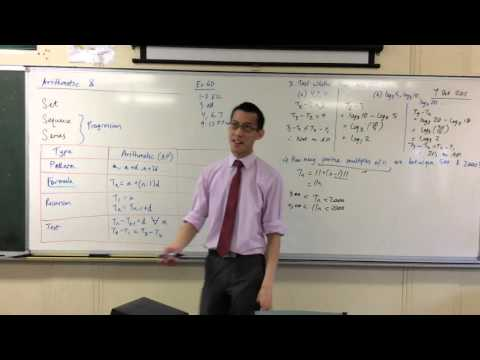Arithmetic Progressions (2 of 2: Testing, Counting Multiples)
3y5m ago
06:09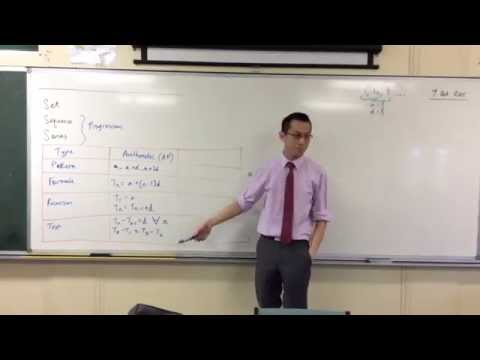Introduction to Arithmetic Progressions (2 of 2: Recursive Definition & Test)
3y5m ago
08:04Introduction to Arithmetic Progressions (1 of 2: Pattern & Formula)
3y5m ago
04:36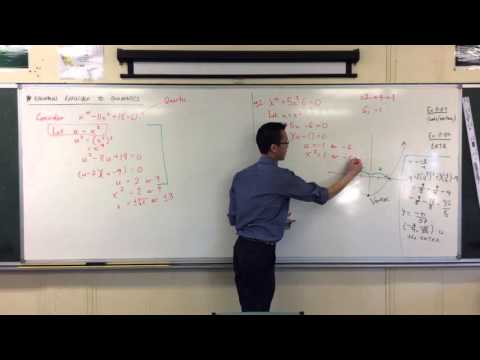Equations Reducible to Quadratics (2 of 2: Extraneous Solutions?)
3y5m ago
06:08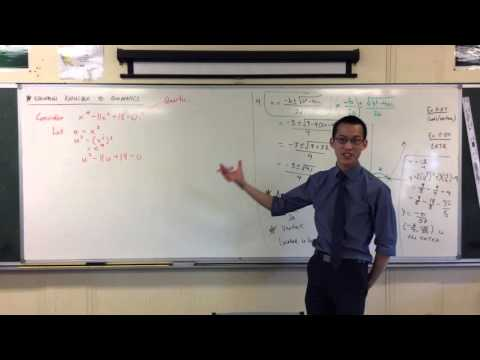Equations Reducible to Quadratics (1 of 2: Introductory Example)
3y5m ago
05:54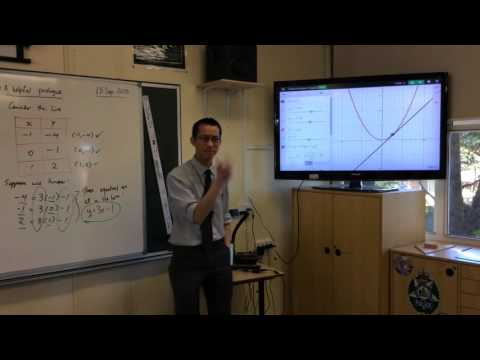Deriving the Chord of Contact (2 of 2: Visual Demonstration)
3y5m ago
05:24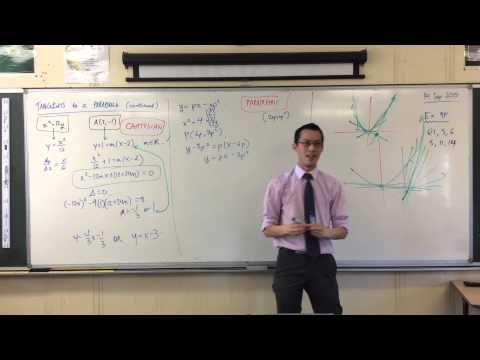Tangents to a Parabola from an External Point (1 of 2: Parametric Approach)
3y5m ago
07:57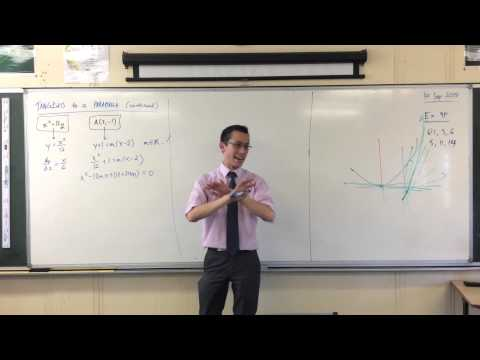Tangents to a Parabola from an External Point (1 of 2: Cartesian Approach)
3y5m ago